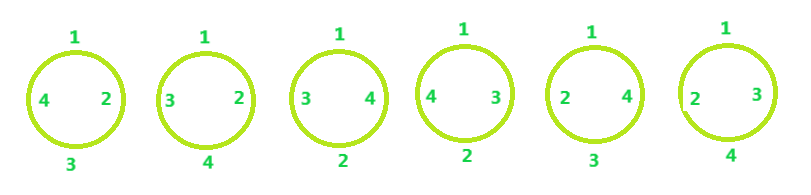Open in App
Not now

# Permutations to arrange N persons around a circular table

• Difficulty Level : Medium
• Last Updated : 31 Aug, 2022

Given N, the number of persons. The task is to arrange N person around a circular table.
Examples

```Input: N = 4
Output: 6

Input: N = 5
Output: 24```

Approach: It is the concept of Circular permutation i.e. there is no specific starting point in the arrangement, any element can be considered as the start of the arrangement.
For N = 4, Arrangements will be:Below is the formula to find Circular permutations:

`Circular Permutations = (N - 1)!`

Below is the implementation of above idea:

## C++

 `// C++ code to demonstrate Circular Permutation``#include ``using` `namespace` `std;` `// Function to find no. of permutations``int` `Circular(``int` `n)``{` `    ``int` `Result = 1;` `    ``while` `(n > 0) {``        ``Result = Result * n;``        ``n--;``    ``}` `    ``return` `Result;``}` `// Driver Code``int` `main()``{``    ``int` `n = 4;` `    ``cout << Circular(n - 1);``}`

## Java

 `// Java code to demonstrate``// Circular Permutation``import` `java.io.*;` `class` `GFG``{``// Function to find no.``// of permutations``static` `int` `Circular(``int` `n)``{` `    ``int` `Result = ``1``;` `    ``while` `(n > ``0``)``    ``{``        ``Result = Result * n;``        ``n--;``    ``}` `    ``return` `Result;``}` `// Driver Code``public` `static` `void` `main(String[] args)``{``    ``int` `n = ``4``;``    ` `    ``System.out.println(Circular(n - ``1``));``}``}` `// This code is contributed``// by Naman_Garg`

## Python 3

 `# Python 3 Program to demonstrate Circular Permutation ` `# Function to find no. of permutations``def` `Circular(n) :``    ``Result ``=` `1` `    ``while` `n > ``0` `:``        ``Result ``=` `Result ``*` `n``        ``n ``-``=` `1` `    ``return` `Result``    ` `# Driver Code``if` `__name__ ``=``=` `"__main__"` `:``    ``n ``=` `4` `    ``# function calling``    ``print``(Circular(n``-``1``))` `# This code is contributed by ANKITRAI1`

## C#

 `// C# code to demonstrate``// Circular Permutation``using` `System;``                    `  `public` `class` `GFG {` `// Function to find no.``// of permutations``static` `int` `Circular(``int` `n)``{`` ` `    ``int` `Result = 1;`` ` `    ``while` `(n > 0)``    ``{``        ``Result = Result * n;``        ``n--;``    ``}`` ` `    ``return` `Result;``}`` ` `// Driver Code``public` `static` `void` `Main()``{``    ``int` `n = 4;``     ` `    ``Console.Write(Circular(n - 1));``}``}`` ` `/* This Java code is contributed by 29AjayKumar*/`

## PHP

 ` 0)``    ``{``        ``\$Result` `= ``\$Result` `* ``\$n``;``        ``\$n``--;``    ``}` `    ``return` `\$Result``;``}` `// Driver Code``\$n` `= 4;` `echo` `Circular(``\$n` `- 1);` `// This code is contributed by mits``?>`

## Javascript

 ``

Output:

`6`

Time Complexity: O(N) where N is the number of persons.
Auxiliary Space: O(1), since no extra space has been taken.

My Personal Notes arrow_drop_up